RS Aggarwal Class 10 Solutions Chapter 19 - Volume And Surface Areas Of Solids Ex 19A (19.1)

RS Aggarwal Class 10 Chapter 19 - Volume And Surface Areas Of Solids Ex 19A (19.1) Solutions Free PDF

Q.1: A medicine capsule is in the shape of a cylinder with hemispherical struck to each of its end. The length of the entire capsule is 14 mm and the diameter of the capsule is 5 mm. Find the surface area.

Sol: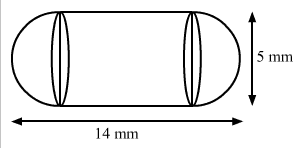We have,

The total height of the capsule = 14 mm and

The radius of the capsule, r = 5/2 mm

Also, the height of the cylinder, h = 14 – (2 × 5/2) = 14 – 5 = 9 mm

Now, the surface area of the capsule = CSA of the cylinder + 2 × CSA of a hemisphere

= 2πrh + 2 × 2πr2

= 2πr (h + 2r)

= 2 × 22/7 × 5/2 × (9 + 2 × 5/2)

= 22/7 × 5 × (9 + 5)

= 22/7 × 5 × 14

= 220 mm2

So, the surface area of the medicine capsule is 220 mm2.

Q.2: A Wooden article was made by scooping out a hemisphere from each end of the cylinder, as shown in the figure. If the height of the cylinder is 20 cm and its base is of diameter 7 cm, find the total surface area of the article when it is ready.

Sol: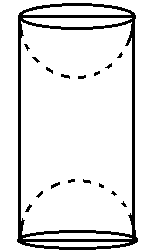Height of cylinder = 20 cm

And diameter = 7 cm and then radius = 3.5 cm

Total surface area of article

= (lateral surface of cylinder with r = 3.5 cm and h = 20 cm)

= [2?rh + 2(2?r2)] sq.units

= $[(2 \times \frac{22}{7} \frac{7}{2} \times 20) \, + \, (4 \times \frac{22}{7} \times \frac{7}{2} \times \frac{7}{2} )] cm^{2}$

= (440 + 154) cm2 = 594 cm2

Q.3: A solid is in the form of a right circular cone mounted on a hemisphere. The radius of the hemisphere is 2.1 cm and the height of the cone is 4 cm. The solid is placed in a cylindrical tube full of water in such a way that the whole solid is submerged in water. If the radius of the cylinder is 5 cm and its height is 9.8 cm, find the volume of the water left in the tub.

Sol: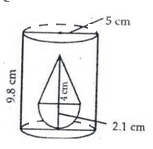Radius of cylinder r1 = 5 cm

And height of cylinder h1 = 9.8 cm

Radius of cone r = 2.1 cm

And the height of cone h2 = 4 cm

Volume of water left in tub = (volume of cylindrical tub – volume of solid)

= $\pi r_{1}^{2} h_{1} – \frac{2}{3} \pi r^{3} – \frac{1}{3} \pi r^{2} h_{2}$

= $\frac{22}{7} \times 5 \times 5 \times 9.8 – \frac{2}{3} \times \frac{22}{7} \times 2.1 \times 2.1 \times 2.1 – \frac{1}{3} \times \frac{22}{7} \times 2.1 \times 2.1 \times 4$

= [(770 – 19.404) – 18.48] cm= 732.116 cm3

Q.4: From a solid cylinder whose height is 8 cm and radius is 6 cm , sa conical cavity of height 8 cm and of base radius 6 cm is hollowed out. Find the volume of the remaining solid. Also find the total surface area of the remaining solid.

Sol: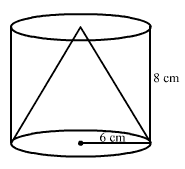Volume of solid left = volume of cylinder – volume of cone

= πr2h – 1/3 πr2h = 2/3 × 22/7 × 8 × 6 × 6 = 603.428 cm3

The slant length of the cone, $l = \sqrt {r^ {2} + h^ {2}} = \sqrt{36 + 64} = 10\; cm$

Total surface of the final solid = area of base circle + curved surface area of cylinder + curved surface area of cone

= $\pi r^ {2} + 2 \pi r h + \pi r l = \pi r \left (r + 2h + l \right ) = \frac {22}{7} \times 6 \times \left ( 6 + 16 + 10 \right ) = 603.42 cm^{2}$

Q.5: From a solid cylinder of height 2.8 cm and diameter 4.2 cm, a conical cavity of the same height and same diameter is hollowed out. Find the total surface area of the remaining solid.

Sol:

The height of the cone = the height of the cylinder = h = 2.8 cm and the radius of the base, r = 4.2/2 = 2.1 cm

The slant height of the cone, $l = \sqrt {r^ {2} + h^{2}}$

$= \sqrt {2.1^ {2} + 2.8^ {2}}$

$= \sqrt {4.41 + 7.84}$

$= \sqrt {12.25}$

= 3.5 cm

Now, the total surface area of the remaining solid = CSA of cylinder + CSA of cone + Area of a base

= 2πrh + πrl + πr2

= πr (2h + l + r)

= 22/7 × 2.1 × (2 × 2.8 + 3.5 + 2.1)

= 22 × 0.3 × (5.6 + 5.6) = 73.92 cm2

So, the total surface area of the remaining solid is 73.92 cm2

Q.6: From a solid cylinder of height 14 cm and base diameter 7 cm, two equal conical holds each of radius 2.1 cm and height 4 cm are cut off. Find the volume of the remaining solid.

Sol:

We have,

The height of the cylinder, H = 14 cm,

The base radius of the cylinder, R = 7/2 cm

The base radius of each conical holes, r = 2.1 cm and the height of each conical holes, h = 4 cm

Volume of the remaining solid = volume of cylinder – volume of 2 conical holes

= πR2H – 2 × 1/3 πr2h

= 22/7 × 7/2 × 7/2 × 14 – 2/3 × 22/7 ×2.1 ×2.1 ×4

= 539 – 36.96 = 502.04 cm3

So, the volume of the remaining solid is 502.04 cm3

Q.7: A metallic cylinder has radius 3 cm and height 5 cm. To reduce its weight, a conical hole is drilled in the cylinder. The conical hole has a radius of $\frac{3}{2}$ cm and its depth is $\frac{8}{9}$ cm. Calculate the ratio of the volume of the metal left in the cylinder to the volume of the metal taken out of conical shape.

Sol:

We have,

The base radius of the cylinder, R = 3 cm,

The height of the cylinder, H = 5 cm,

The base radius of the conical hole, r = 3/2 cm and

The height of the conical hole, h = 8/9 cm

Now,

Volume of the cylinder, V = πR2H

= π × 32 × 5 = 45π cm3

Also,

Volume of the cone removed from the cylinder, v = 1/3 πr2h

$\frac {\pi } {3} \times \left ( \frac {3} {2} \right )^ {2} \times \left ( \frac{8} {9} \right )$

= $\frac {2 \pi } {3} cm^ {3}$

So, the volume of metal left in cylinder, V’ = V – v

= $45 \pi – \frac {2 \pi } {3}$

= $= \frac {133 \pi } {3} cm^ {3}$

Therefore, the required ratio = $\frac {V’} {v}$

$\frac { \left ( \frac {133\pi } {3} \right )} { \left ( \frac {2 \pi } {3} \right )}$

$\frac {133} {2}$ = 133:2

So, the ratio of the volume of metal left in cylinder to the volume to the volume of metal taken out in conical shape is 133:2

Q.8: A spherical glass vessel has a cylinder neck 7 cm long and 4 cm in diameter. The diameter of the spherical part is 21 cm. Find the quantity of water it can hold.  [Take $\pi = \frac{22}{7}$ ]

Sol: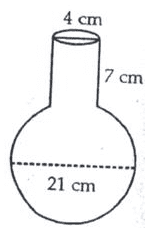Diameter of the spherical part of vessel = 21 cm

Its volume = $\frac{4}{3} \pi r^{3}$

= $\frac{4}{3} \times \frac{22}{7} \times \frac{21}{2} \times \frac{21}{2} \times \frac{21}{2}$ = 11(21)2 cm3 = 4851 cm3

Volume of cylindrical part of vessel

= $\pi r^{2} h = \frac{22}{7} \times 2 \times 2 \times 7 cm^{3}$

= 88 cm3

Volume of whole vessel = (4851 + 88) cm3 = 4939 cm3

Q.9: From a cubical piece of wood of side 21 cm a hemisphere is carved out in such a way that the diameter of the hemisphere is equal to the side of the cubicle piece. Find the surface area and volume of the remaining piece.

Sol: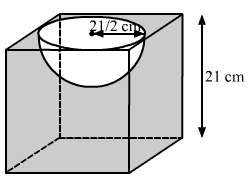We have, The edge of the cubical piece, a = 21 cm and

The radius of the hemisphere, r = a/2 = 21/2 cm

The surface area of the remaining piece = TSA of cube + CSA of hemisphere – Area of circle

= 6a2 + 2 πr2 – πr2

= 6a2 + πr2

= 6 × 21 × 21 + 22/7 × 21/2 × 21/2

= $21 \times 21 \left (6 + \frac {22} {7 \times 4} \right)$

= $21 \times 21 \left (6 + \frac{11} {14} \right)$

= $21 \times 21 \left (\frac{84 + 11} {14} \right)$

= $21 \times 3 \left (\frac{95} {2} \right)$

= 2992.5 cm2

Also

Volume of remaining piece = volume of cube – volume of the hemisphere

= a3 – 2/3 πr3

= $21 \times 21 \times 21 – \frac{2} {3} \times \frac {22} {7} \times \left (\frac {21} {2} \right) \times \left (\frac {21} {2} \right) \times \left (\frac{21}{2} \right)$

= $21 \times 21 \times 21 \times \left (1 – \frac {2} {3} \times \frac {22} {7} \times \frac {1} {2} \times \frac {1} {2} \times \frac {1} {2} \right )$

= $21 \times 21 \times 21 \times \left ( \frac {1} {1} – \frac {11} {42} \right )$

= $21 \times 21 \times 21 \times \left ( \frac {42 – 11} {42} \right )$

= $21 \times 21 \times \left ( \frac {31} {2} \right )$ = 6835.5 cm3

Q.10: A toy is in the shape of a right circular cylinder with the hemisphere on one end and a cone on the other. The radius and the height of the cylinder part are 5 cm and 13 cm respectively. The radii of the hemispherical and the conical parts are the same as that of the cylindrical part. Find the surface area of the toy, if the total height of the toy is 30 cm

Sol: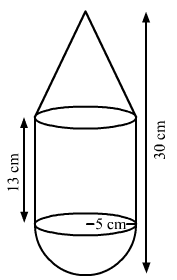We have,

The base radius of the cone = the base radius of the cylinder = the base radius of the hemisphere = r = 5 cm,

The height of the cylinder, H = 13 cm,

The total height of the toy = 30 cm

Also, the height of the cone, h = 30 – (13 + 5) = 12 cm

The slant height of the cone, $l = \sqrt {r^{2} + h^ {2} }$

= $\sqrt {5^ {2} + 12^ {2} }$

= $\sqrt {25 + 144}$

= $\sqrt {169}$

= 13 cm

Now, the surface area of the toy = CSA of cone + CSA of cylinder + CSA of hemisphere

= πrl + 2 πrH + 2 πr2

= πr (l + 2H +2r)

= 22/7 × 5 × (13 + 2 × 13 + 2 × 5)

= 22/7 × 5 × (13 + 26 + 10)

= 22/7 × 5 × 49

= 770 cm2

So, the surface area of the toy is 770 cm2

Q.11: The inner diameter of a glass is 7 cm and it has a raised portion in the bottom in the shape of a hemisphere, as shown in the figure. If the height of the glass is 16 cm, find the apparent capacity and actual capacity of the glass.

Sol: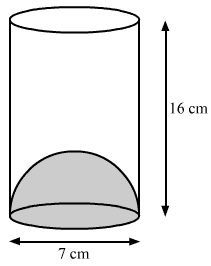We have,

The height of the glass, h = 16 cm and

The base radius of the cylinder = the base radius of the hemisphere, r = 7/2 cm

Now,

The apparent capacity of the glass = Volume of the cylinder

= πr2h

= $\frac {22} {7} \times \frac {7} {2} \times \frac {7} {2} \times 16$

We have,

The height of the glass, h = 16 cm and

The base radius of the cylinder = the base radius of the hemisphere, r = 7/2 cm

Now,

The apparent capacity of the glass = Volume of the cylinder

= πr2h

= $\frac {22} {7} \times \frac {7} {2} \times \frac {7} {2} \times 16 \]”> = 616 cm3 Also, The actual capacity of the glass = Volume of cylinder – Volume of hemisphere = \( 616 – \frac {2} {3} \pi r^ {3}$

= $616 – \frac {2} {3} \times \frac {22} {7} \times \frac {7} {2} \times \frac {7} {2}\times \frac {7} {2}$

= $616 – \frac {539}{6}$

= $\frac {3157} {6}$

= 526. 17 cm3

Q.12: A wooden toy is in the shape of a cone mounted on a cylinder, as shown in the figure. The total height of the tower is 26 cm while the height of the conical part is 6 cm. The diameter of the base of the conical part is 5 cm and that of the cylindrical part is 4 cm. The conical part and the cylindrical part are respectively painted red and white. Find the area to be painted by each of the colours. [ Take $\pi = \frac{22}{7}$ ]

Sol: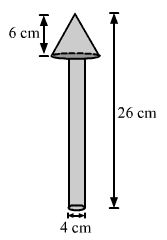We have,

The base radius of the conical part, r = 5/2 = 2.5 cm,

The base radius of the cylindrical part, R = 4/2 = 2 cm,

The total height of the toy = 26 cm,

The height of the conical part, h = 6 cm

Also,

The height of the cylindrical part, H = 26 – 6 = 20 cm

And, the slant height of the conical part, $l = \sqrt {r^ {2} + h^ {2}} = \sqrt {2.5^ {2} + 6^ {2}} = \sqrt {6.25 + 36} = \sqrt {42.25} = 6.5 \; cm$

Now,

The area to be painted by the red color = CSA of cone + Area of base of conical part – Area of base of the cylindrical part

= πrl + πr2 – πR2

= $\frac {22} {7} \times 2.5 \times 6.5 + \frac {22} {7} \times 2.5 \times 2.5 – \frac {22} {7} \times 2 \times 2$

= $\frac {22} {7} \times 16.25 + \frac {22} {7} \times 6.5 – \frac {22} {7} \times 4$

= $\frac {22} {7} \times \left (16.25 + 6.5 – 4 \right)$

= $\frac {22} {7} \times 18.5$

= 58.14 cm2

Also,

The area to be painted by white color = CSA of cylinder + Area of base of cylinder

= $2 \pi R H + \pi R^ {2}$

= $\pi R \left (2H + R \right)$

= $\frac {22} {7} \times 2 \times \left ( 2 \times 20 + 2 \right )$

= $\frac{22} {7} \times 2 \times 42$ = 264 cm2

Practise This Question

State true or false.
Suppose a room has a magnetic field, directed downwards. Then in order to experience the maximum magnetic force, the current carrying wire must be kept horizontal for it to be perpendicular to the magnetic field.